•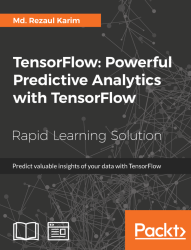#### TensorFlow: Powerful Predictive Analytics with TensorFlow#### Overview of this book

Predictive analytics discovers hidden patterns from structured and unstructured data for automated decision making in business intelligence. Predictive decisions are becoming a huge trend worldwide, catering to wide industry sectors by predicting which decisions are more likely to give maximum results. TensorFlow, Google’s brainchild, is immensely popular and extensively used for predictive analysis. This book is a quick learning guide on all the three types of machine learning, that is, supervised, unsupervised, and reinforcement learning with TensorFlow. This book will teach you predictive analytics for high-dimensional and sequence data. In particular, you will learn the linear regression model for regression analysis. You will also learn how to use regression for predicting continuous values. You will learn supervised learning algorithms for predictive analytics. You will explore unsupervised learning and clustering using K-meansYou will then learn how to predict neighborhoods using K-means, and then, see another example of clustering audio clips based on their audio features. This book is ideal for developers, data analysts, machine learning practitioners, and deep learning enthusiasts who want to build powerful, robust, and accurate predictive models with the power of TensorFlow. This book is embedded with useful assessments that will help you revise the concepts you have learned in this book. This book is repurposed for this specific learning experience from material from Packt's Predictive Analytics with TensorFlow by Md. Rezaul Karim.
TensorFlow: Powerful Predictive Analytics with TensorFlowCreditsPrefaceFree Chapter
From Data to Decisions – Getting Started with TensorFlowPutting Data in Place – Supervised Learning for Predictive AnalyticsClustering Your Data – Unsupervised Learning for Predictive AnalyticsUsing Reinforcement Learning for Predictive Analytics## Getting Started with TensorFlow – Linear Regression and Beyond

In this example, we will take a closer look at TensorFlow's and TensorBoard's main concepts and try to do some basic operations to get you started. The model we want to implement simulates the linear regression.

In the statistics and machine learning realm, linear regression is a technique frequently used to measure the relationship between variables. This is also a quite simple but effective algorithm that can be used in predictive modeling too. Linear regression models the relationship between a dependent variable yi, an interdependent variable xi, and a random term b. This can be seen as follows: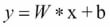Now to conceptualize the preceding equation, I am going to write a simple Python program for creating data into a 2D space. Then I will use TensorFlow to look for the line that best fits in the data points:

```# Import libraries (Numpy, matplotlib)
import numpy as np
import matplotlib.pyplot as plot

# Create 1000 points following a function y=0.1 * x + 0.4 (i.e. y \= W * x + b) with some normal random distribution:

num_points = 1000
vectors_set = []
for i in range(num_points):
W = 0.1 # W
b = 0.4 # b
x1 = np.random.normal(0.0, 1.0)
nd = np.random.normal(0.0, 0.05)
y1 = W * x1 + b

# Add some impurity with some normal distribution -i.e. nd:
y1 = y1+nd

# Append them and create a combined vector set:
vectors_set.append([x1, y1])

# Separate the data point across axises:
x_data = [v for v in vectors_set]
y_data = [v for v in vectors_set]

# Plot and show the data points in a 2D space
plt.plot(x_data, y_data, 'r*', label='Original data')
plt.legend()
plt.show()```

If your compiler does not make any complaints, you should observe the following graph: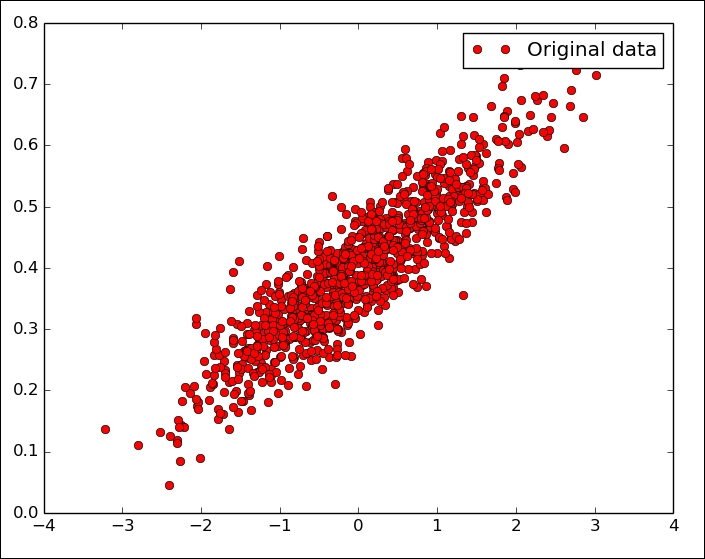Figure 12: Randomly generated (but original) data

Well, so far we have just created a few data points without any associated model that could be executed through TensorFlow. So the next step is to create a linear regression model to be able to obtain the output values `y` that is estimated from the input data points–that is, `x_data`. In this context, we have only two associated parameters–that is, `W` and `b`. Now the objective is to create a graph that allows finding the values for these two parameters based on the input data `x_data` by adjusting them to `y_data`–that is, optimization problem.

So the target function in our case would be as follows: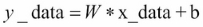If you recall, we defined W = 0.1 and b = 0.4 while creating the data points in the 2D space. Now TensorFlow has to optimize these two values so that `W` tends to 0.1 and `b` to 0.4, but without knowing any optimization function, TensorFlow does not even know anything.

A standard way to solve such optimization problems is to iterate through each value of the data points and adjust the value of `W` and `b` in order to get a more precise answer on each iteration. Now to realize if the values are really improving, we need to define a cost function that measures how good a certain line is.

In our case, the cost function is the mean squared error that helps find the average of the errors based on the distance function between the real data points and the estimated ones on each iteration. We start by importing the TensorFlow library:

```import tensorflow as tf
W = tf.Variable(tf.random_uniform(, -1.0, 1.0))
b = tf.Variable(tf.zeros())
y = W * x_data + b```

In the preceding code segment, we are generating a random point using a different strategy and storing in variable W. Now let's define a loss function loss=mean [(y−y_data) 2] and this returns a scalar value with the mean of all distances between our data and the model prediction. In terms of TensorFlow convention, the loss function can be expressed as follows:

`loss = tf.reduce_mean(tf.square(y - y_data))`

Without going into further detail, we can use some widely used optimization algorithms such as gradient descent. At a minimal level, the gradient descent is an algorithm that works on a set of given parameters that we already have. It starts with an initial set of parameter values and iteratively moves toward a set of values that minimize the function by taking another parameter called learning rate. This iterative minimization is achieved by taking steps in the negative direction of the function called gradient.

```optimizer = tf.train.GradientDescentOptimizer(0.6)
train = optimizer.minimize(loss)```

Before running this optimization function, we need to initialize all the variables that we have so far. Let's do it using TensorFlow convention as follows:

```init = tf.global_variables_initializer()
sess = tf.Session()
sess.run(init)```

Since we have created a TensorFlow session, we are ready for the iterative process that helps us find the optimal values of `W` and `b`:

```for i in range(16):
sess.run(train)
print(i, sess.run(W), sess.run(b), sess.run(loss))```

You should observe the following output:

```>>>
0 [ 0.18418592] [ 0.47198644] 0.0152888
1 [ 0.08373772] [ 0.38146532] 0.00311204
2 [ 0.10470386] [ 0.39876288] 0.00262051
3 [ 0.10031486] [ 0.39547175] 0.00260051
4 [ 0.10123629] [ 0.39609471] 0.00259969
5 [ 0.1010423] [ 0.39597753] 0.00259966
6 [ 0.10108326] [ 0.3959994] 0.00259966
7 [ 0.10107458] [ 0.39599535] 0.00259966```

Thus you can see the algorithm starts with the initial values of W = 0.18418592 and b = 0.47198644 where the loss is pretty high. Then the algorithm iteratively adjusted the values by minimizing the cost function. In the eighth iteration, all the values tend to our desired values.

Now what if we could plot them? Let's do it by adding the plotting line under the `for` loop as follows: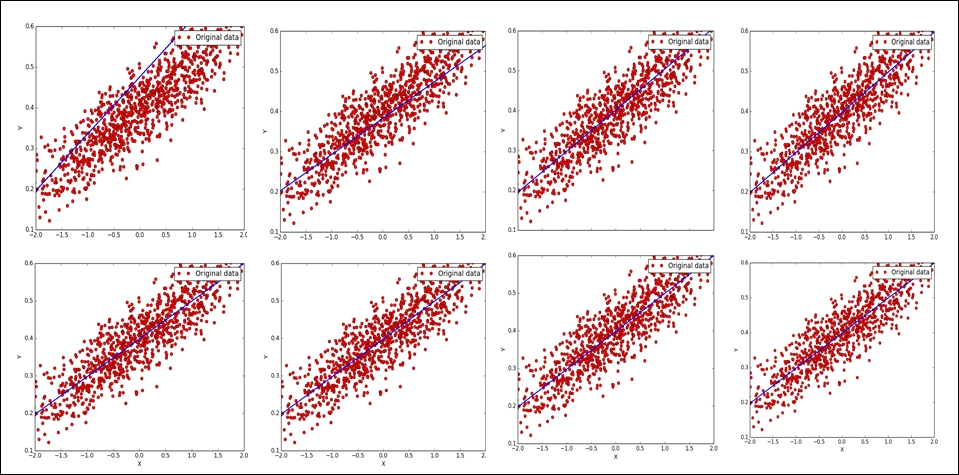Figure 13: Linear regression after eight iteration that optimizes the loss function

Now let's iterate the same up to the 16th iteration:

```>>>
0 [ 0.23306453] [ 0.47967502] 0.0259004
1 [ 0.08183448] [ 0.38200468] 0.00311023
2 [ 0.10253634] [ 0.40177572] 0.00254209
3 [ 0.09969243] [ 0.39778906] 0.0025257
4 [ 0.10008509] [ 0.39859086] 0.00252516
5 [ 0.10003048] [ 0.39842987] 0.00252514
6 [ 0.10003816] [ 0.39846218] 0.00252514
7 [ 0.10003706] [ 0.39845571] 0.00252514
8 [ 0.10003722] [ 0.39845699] 0.00252514
9 [ 0.10003719] [ 0.39845672] 0.00252514
10 [ 0.1000372] [ 0.39845678] 0.00252514
11 [ 0.1000372] [ 0.39845678] 0.00252514
12 [ 0.1000372] [ 0.39845678] 0.00252514
13 [ 0.1000372] [ 0.39845678] 0.00252514
14 [ 0.1000372] [ 0.39845678] 0.00252514
15 [ 0.1000372] [ 0.39845678] 0.00252514```

Much better and we're closer to the optimized values, right? Now, what if we further improve our visual analytics through TensorFlow that help visualize what is happening in these graphs. TensorBoard provides a web page for debugging your graph as well as inspecting the used variables, node, edges, and their corresponding connections.

However, to get the facility of the preceding regression analysis, you need to annotate the preceding graphs with the variables such as loss function, `W`, `b`, `y_data`, `x_data`, and so on. Then you need to generate all the summaries by invoking the function `tf.summary.merge_all()`.

Now, we need to make the following changes to the preceding code. However, it is a good practice to group related nodes on the graph using the `tf.name_scope()` function. Thus, we can use `tf.name_scope()` to organize things on the TensorBoard graph view, but let's give it a better name:

```with tf.name_scope("LinearRegression") as scope:
W = tf.Variable(tf.random_uniform(, -1.0, 1.0), name="Weights")
b = tf.Variable(tf.zeros())y = W * x_data + b```

Then let's annotate the loss function in a similar way, but by giving a suitable name such as `LossFunction`:

```with tf.name_scope("LossFunction") as scope:
loss = tf.reduce_mean(tf.square(y - y_data))```

Let's annotate the loss, weights, and bias that are needed for the TensorBoard:

```loss_summary = tf.summary.scalar("loss", loss)
w_ = tf.summary.histogram("W", W)
b_ = tf.summary.histogram("b", b)```

Well, once you annotate the graph, it's time to configure the summary by merging them:

`merged_op = tf.summary.merge_all()`

Now before running the training (after the initialization), write the summary using the `tf.summary.FileWriter()` API as follows:

`writer_tensorboard = tf.summary.FileWriter('/home/asif/LR/', sess.graph_def)`

Then start the TensorBoard as follows:

```\$ tensorboard –logdir=<trace_file_name>
```

In our case, it could be something like the following:

```\$ tensorboard --logdir=/home/asif/LR/
```

Now let's move to `http://localhost:6006` and on clicking on the GRAPHS tab, you should see the following graph: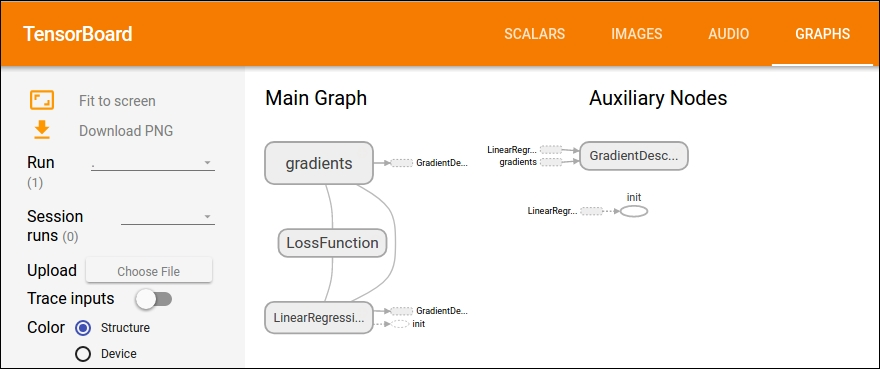Figure 14: Main graph and auxiliary nodes on TensorBoard

### Source Code for the Linear Regression

We reported for the entire source code for the example previously described:

```# Import libraries (Numpy, Tensorflow, matplotlib)
import numpy as np
import matplotlib.pyplot as plot

# Create 1000 points following a function y=0.1 * x + 0.4 (i.e. y = W * x + b) with some normal random distribution:
num_points = 1000
vectors_set = []
for i in range(num_points):
W = 0.1  # W
b = 0.4  # b
x1 = np.random.normal(0.0, 1.0)
nd = np.random.normal(0.0, 0.05)
y1 = W * x1 + b

# Add some impurity with some normal distribution -i.e. nd:y1 = y1 + nd

# Append them and create a combined vector set:
vectors_set.append([x1, y1])

# Separate the data point across axises
x_data = [v for v in vectors_set]
y_data = [v for v in vectors_set]

# Plot and show the data points in a 2D space
plot.plot(x_data, y_data, 'ro', label='Original data')
plot.legend()
plot.show()

import tensorflow as tf

#tf.name_scope organize things on the tensorboard graph view
with tf.name_scope("LinearRegression") as scope:
W = tf.Variable(tf.random_uniform(, -1.0, 1.0), name="Weights")
b = tf.Variable(tf.zeros())
y = W * x_data + b

# Define a loss function that takes into account the distance between the prediction and our dataset
with tf.name_scope("LossFunction") as scope:
loss = tf.reduce_mean(tf.square(y - y_data))

train = optimizer.minimize(loss)

# Annotate loss, weights, and bias (Needed for tensorboard)
loss_summary = tf.summary.scalar("loss", loss)
w_ = tf.summary.histogram("W", W)
b_ = tf.summary.histogram("b", b)

# Merge all the summaries
merged_op = tf.summary.merge_all()

init = tf.global_variables_initializer()
sess = tf.Session()
sess.run(init)

# Writer for TensorBoard  (replace with our preferred location
writer_tensorboard = tf.summary.FileWriter('/ LR/', sess.graph_def)

for i in range(16):
sess.run(train)
print(i, sess.run(W), sess.run(b), sess.run(loss))
plot.plot(x_data, y_data, 'ro', label='Original data')
plot.plot(x_data, sess.run(W)*x_data + sess.run(b))
plot.xlabel('X')
plot.xlim(-2, 2)
plot.ylim(0.1, 0.6)
plot.ylabel('Y')
plot.legend()
plot.show()
# Finally, close the TensorFlow session when you're done
sess.close()```

Ubuntu may ask you to install the python-tk package. You can do it by executing the following command on Ubuntu:

```\$ sudo apt-get install python-tk
# For Python 3.x, use the following
\$ sudo apt-get install python3-tk
```